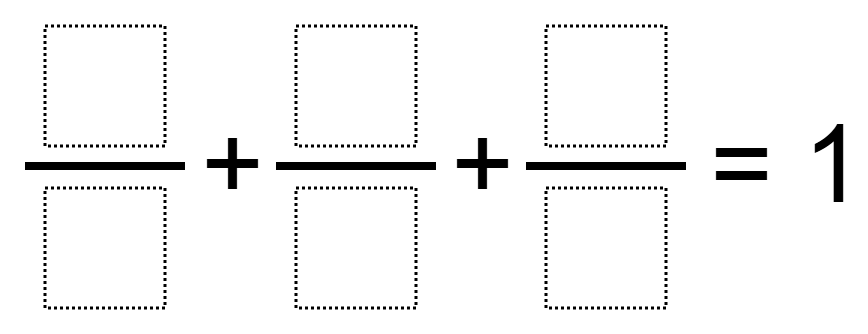Home > Grade 5 > Adding Fractions 4

# Adding Fractions 4

Directions: Using the integers 1 to 10 at most one time each, fill in the boxes so that the sum is equal to 1.### Hint

What three unit fractions add up to exactly 1 whole? What numbers would you use for the denominators? Why?

### Answer

There are many solutions. Here are some possible solutions:
1/6 + 4/8 + 3/9
3/6 + 2/8 + 1/4

Source: Joshua Nelson

## Multiplying Decimals to Make a Whole Number Product

Directions: Using the digits 1 to 9 at most one time each, fill in the …

### One comment

1.Our class came up with two different solutions:

1.) 1/4 + 2/8 + 5/10

2.) 3/6 + 1/4 + 2/8